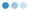# Sangeeta khareSoftware developer

MessagePhoneFdgdfgfdgdfffffffffffffffffffffff fdgddddddddddddddddddddddddddddddddddddddddddddddddddddddddddddddddddddddddddddddddddddddddddddddddddddddddddddddddd fdgdfgfdgdfffffffffffffffffffffff fdgdddddddddddddddddddddddddddddddddddddddddddddddddddddddddddddddddddddddddddddddddddddddddddddddddddddddddddddddddddddddddddddddddddddddddgfdgdgdg fdgdfgfdgdfffffffffffffffffffffff fdgdddddddddddddddddddddddddddddddddddddddddddddddddddddddddddddddddddddddddddddddddddddddddddddddddddddddddddddddddddddddddddddddddddddddddgfdgdgdg fdgdfgfdgdfffffffffffffffffffffff fdgdddddddddddddddddddddddddddddddddddddddddddddddddddddddddddddddddddddddddddddddddddddddddddddddddddddddddddddddddddddddddddddddddddddddddgfdgdgdg fdgdfgfdgdfffffffffffffffffffffff fdgdddddddddddddddddddddddddddddddddddddddddddddddddddddddddddddddddddddddddddddddddddddddddddddddddddddddddddddddddddddddddddddddddddddddddgfdgdgdg fdgdfgfdgdfffffffffffffffffffffff fdgdddddddddddddddddddddddddddddddddddddddddddddddddddddddddddddddddddddddddddddddddddddddddddddddddddddddddddddddddddddddddddddddddddddddddgfdgdgdg fdgdfgfdgdfffffffffffffffffffffff fdgdddddddddddddddddddddddddddddddddddddddddddddddddddddddddddddddddddddddddddddddddddddddddddddddddddddddddddddddddddddddddddddddddddddddddgfdgdgdg fdgdfgfdgdfffffffffffffffffffffff fdgdddddddddddddddddddddddddddddddddddddddddddddddddddddddddddddddddddddddddddddddddddddddddddddddddddddddddddddddddddddddddddddddddddddddddgfdgdgdg fdgdfgfdgdfffffffffffffffffffffff fdgdddddddddddddddddddddddddddddddddddddddddddddddddddddddddddddddddddddddddddddddddddddddddddddddddddddddddddddddddddddddddddddddddddddddddgfdgdgdg fdgdfgfdgdfffffffffffffffffffffff fdgdddddddddddddddddddddddddddddddddddddddddddddddddddddddddddddddddddddddddddddddddddddddddddddddddddddddddddddddddddddddddddddddddddddddddgfdgdgdg fdgdfgfdgdfffffffffffffffffffffff fdgdddddddddddddddddddddddddddddddddddddddddddddddddddddddddddddddddddddddddddddddddddddddddddddddddddddddddddddddddddddddddddddddddddddddddgfdgdgdg fdgdfgfdgdfffffffffffffffffffffff fdgdddddddddddddddddddddddddddddddddddddddddddddddddddddddddddddddddddddddddddddddddddddddddddddddddddddddddddddddddddddddddddddddddddddddddgfdgdgdg fdgdfgfdgdfffffffffffffffffffffff fdgdddddddddddddddddddddddddddddddddddddddddddddddddddddddddddddddddddddddddddddddddddddddddddddddddddddddddddddddddddddddddddddddddddddddddgfdgdgdg fdgdfgfdgdfffffffffffffffffffffff fdgdddddddddddddddddddddddddddddddddddddddddddddddddddddddddddddddddddddddddddddddddddddddddddddddddddddddddddddddddddddddddddddddddddddddddgfdgdgdg fdgdfgfdgdfffffffffffffffffffffff fdgdddddddddddddddddddddddddddddddddddddddddddddddddddddddddddddddddddddddddddddddddddddddddddddddddddddddddddddddddddddddddddddddddddddddddgfdgdgdg fdgdfgfdgdfffffffffffffffffffffff fdgdddddddddddddddddddddddddddddddddddddddddddddddddddddddddddddddddddddddddddddddddddddddddddddddddddddddddddddddddddddddddddddddddddddddddgfdgdgdg fdgdfgfdgdfffffffffffffffffffffff fdgdddddddddddddddddddddddddddddddddddddddddddddddddddddddddddddddddddddddddddddddddddddddddddddddddddddddddddddddddddddddddddddddddddddddddgfdgdgdg fdgdfgfdgdfffffffffffffffffffffff fdgdddddddddddddddddddddddddddddddddddddddddddddddddddddddddddddddddddddddddddddddddddddddddddddddddddddddddddddddddddddddddddddddddddddddddgfdgdgdg fdgdfgfdgdfffffffffffffffffffffff fdgdddddddddddddddddddddddddddddddddddddddddddddddddddddddddddddddddddddddddddddddddddddddddddddddddddddddddddddddddddddddddddddddddddddddddgfdgdgdg fdgdfgfdgdfffffffffffffffffffffff fdgdddddddddddddddddddddddddddddddddddddddddddddddddddddddddddddddddddddddddddddddddddddddddddddddddddddddddddddddddddddddddddddddddddddddddgfdgdgdg fdgdfgfdgdfffffffffffffffffffffff fdgdddddddddddddddddddddddddddddddddddddddddddddddddddddddddddddddddddddddddddddddddddddddddddddddddddddddddddddddddddddddddddddddddddddddddgfdgdgdg fdgdfgfdgdfffffffffffffffffffffff fdgdddddddddddddddddddddddddddddddddddddddddddddddddddddddddddddddddddddddddddddddddddddddddddddddddddddddddddddddddddddddddddddddddddddddddgfdgdgdg fdgdfgfdgdfffffffffffffffffffffff fdgdddddddddddddddddddddddddddddddddddddddddddddddddddddddddddddddddddddddddddddddddddddddddddddddddddddddddddddddddddddddddddddddddddddddddgfdgdgdg fdgdfgfdgdfffffffffffffffffffffff fdgdddddddddddddddddddddddddddddddddddddddddddddddddddddddddddddddddddddddddddddddddddddddddddddddddddddddddddddddddddddddddddddddddddddddddgfdgdgdg fdgdfgfdgdfffffffffffffffffffffff fdgdddddddddddddddddddddddddddddddddddddddddddddddddddddddddddddddddddddddddddddddddddddddddddddddddddddddddddddddddddddddddddddddddddddddddgfdgdgdg fdgdfgfdgdfffffffffffffffffffffff fdgdddddddddddddddddddddddddddddddddddddddddddddddddddddddddddddddddddddddddddddddddddddddddddddddddddddddddddddddddddddddddddddddddddddddddgfdgdgdg fdgdfgfdgdfffffffffffffffffffffff fdgdddddddddddddddddddddddddddddddddddddddddddddddddddddddddddddddddddddddddddddddddddddddddddddddddddddddddddddddddddddddddddddddddddddddddgfdgdgdg fdgdfgfdgdfffffffffffffffffffffff fdgdddddddddddddddddddddddddddddddddddddddddddddddddddddddddddddddddddddddddddddddddddddddddddddddddddddddddddddddddddddddddddddddddddddddddgfdgdgdg fdgdfgfdgdfffffffffffffffffffffff fdgdddddddddddddddddddddddddddddddddddddddddddddddddddddddddddddddddddddddddddddddddddddddddddddddddddddddddddddddddddddddddddddddddddddddddgfdgdgdg fdgdfgfdgdfffffffffffffffffffffff fdgdddddddddddddddddddddddddddddddddddddddddddddddddddddddddddddddddddddddddddddddddddddddddddddddddddddddddddddddddddddddddddddddddddddddddgfdgdgdg fdgdfgfdgdfffffffffffffffffffffff fdgdddddddddddddddddddddddddddddddddddddddddddddddddddddddddddddddddddddddddddddddddddddddddddddddddddddddddddddddddddddddddddddddddddddddddgfdgdgdg fdgdfgfdgdfffffffffffffffffffffff fdgdddddddddddddddddddddddddddddddddddddddddddddddddddddddddddddddddddddddddddddddddddddddddddddddddddddddddddddddddddddddddddddddddddddddddgfdgdgdg fdgdfgfdgdfffffffffffffffffffffff fdgdddddddddddddddddddddddddddddddddddddddddddddddddddddddddddddddddddddddddddddddddddddddddddddddddddddddddddddddddddddddddddddddddddddddddgfdgdgdg fdgdfgfdgdfffffffffffffffffffffff fdgdddddddddddddddddddddddddddddddddddddddddddddddddddddddddddddddddddddddddddddddddddddddddddddddddddddddddddddddddddddddddddddddddddddddddgfdgdgdg fdgdfgfdgdfffffffffffffffffffffff fdgdddddddddddddddddddddddddddddddddddddddddddddddddddddddddddddddddddddddddddddddddddddddddddddddddddddddddddddddddddddddddddddddddddddddddgfdgdgdg fdgdfgfdgdfffffffffffffffffffffff fdgdddddddddddddddddddddddddddddddddddddddddddddddddddddddddddddddddddddddddddddddddddddddddddddddddddddddddddddddddddddddddddddddddddddddddgfdgdgdg fdgdfgfdgdfffffffffffffffffffffff fdgdddddddddddddddddddddddddddddddddddddddddddddddddddddddddddddddddddddddddddddddddddddddddddddddddddddddddddddddddddddddddddddddddddddddddgfdgdgdg fdgdfgfdgdfffffffffffffffffffffff fdgdddddddddddddddddddddddddddddddddddddddddddddddddddddddddddddddddddddddddddddddddddddddddddddddddddddddddddddddddddddddddddddddddddddddddgfdgdgdg fdgdfgfdgdfffffffffffffffffffffff fdgdddddddddddddddddddddddddddddddddddddddddddddddddddddddddddddddddddddddddddddddddddddddddddddddddddddddddddddddddddddddddddddddddddddddddgfdgdgdg fdgdfgfdgdfffffffffffffffffffffff fdgdddddddddddddddddddddddddddddddddddddddddddddddddddddddddddddddddddddddddddddddddddddddddddddddddddddddddddddddddddddddddddddddddddddddddgfdgdgdg fdgdfgfdgdfffffffffffffffffffffff fdgdddddddddddddddddddddddddddddddddddddddddddddddddddddddddddddddddddddddddddddddddddddddddddddddddddddddddddddddddddddddddddddddddddddddddgfdgdgdg fdgdfgfdgdfffffffffffffffffffffff fdgdddddddddddddddddddddddddddddddddddddddddddddddddddddddddddddddddddddddddddddddddddddddddddddddddddddddddddddddddddddddddddddddddddddddddgfdgdgdg fdgdfgfdgdfffffffffffffffffffffff fdgdddddddddddddddddddddddddddddddddddddddddddddddddddddddddddddddddddddddddddddddddddddddddddddddddddddddddddddddddddddddddddddddddddddddddgfdgdgdg fdgdfgfdgdfffffffffffffffffffffff fdgdddddddddddddddddddddddddddddddddddddddddddddddddddddddddddddddddddddddddddddddddddddddddddddddddddddddddddddddddddddddddddddddddddddddddgfdgdgdg fdgdfgfdgdfffffffffffffffffffffff fdgdddddddddddddddddddddddddddddddddddddddddddddddddddddddddddddddddddddddddddddddddddddddddddddddddddddddddddddddddddddddddddddddddddddddddgfdgdgdg fdgdfgfdgdfffffffffffffffffffffff fdgdddddddddddddddddddddddddddddddddddddddddddddddddddddddddddddddddddddddddddddddddddddddddddddddddddddddddddddddddddddddddddddddddddddddddgfdgdgdg fdgdfgfdgdfffffffffffffffffffffff fdgdddddddddddddddddddddddddddddddddddddddddddddddddddddddddddddddddddddddddddddddddddddddddddddddddddddddddddddddddddddddddddddddddddddddddgfdgdgdg fdgdfgfdgdfffffffffffffffffffffff fdgdddddddddddddddddddddddddddddddddddddddddddddddddddddddddddddddddddddddddddddddddddddddddddddddddddddddddddddddddddddddddddddddddddddddddgfdgdgdg fdgdfgfdgdfffffffffffffffffffffff fdgdddddddddddddddddddddddddddddddddddddddddddddddddddddddddddddddddddddddddddddddddddddddddddddddddddddddddddddddddddddddddddddddddddddddddgfdgdgdg fdgdfgfdgdfffffffffffffffffffffff fdgdddddddddddddddddddddddddddddddddddddddddddddddddddddddddddddddddddddddddddddddddddddddddddddddddddddddddddddddddddddddddddddddddddddddddgfdgdgdg fdgdfgfdgdfffffffffffffffffffffff fdgdddddddddddddddddddddddddddddddddddddddddddddddddddddddddddddddddddddddddddddddddddddddddddddddddddddddddddddddddddddddddddddddddddddddddgfdgdgdg fdgdfgfdgdfffffffffffffffffffffff fdgdddddddddddddddddddddddddddddddddddddddddddddddddddddddddddddddddddddddddddddddddddddddddddddddddddddddddddddddddddddddddddddddddddddddddgfdgdgdg fdgdfgfdgdfffffffffffffffffffffff fdgdddddddddddddddddddddddddddddddddddddddddddddddddddddddddddddddddddddddddddddddddddddddddddddddddddddddddddddddddddddddddddddddddddddddddgfdgdgdg fdgdfgfdgdfffffffffffffffffffffff fdgdddddddddddddddddddddddddddddddddddddddddddddddddddddddddddddddddddddddddddddddddddddddddddddddddddddddddddddddddddddddddddddddddddddddddgfdgdgdg fdgdfgfdgdfffffffffffffffffffffff fdgdddddddddddddddddddddddddddddddddddddddddddddddddddddddddddddddddddddddddddddddddddddddddddddddddddddddddddddddddddddddddddddddddddddddddgfdgdgdg ddddddddddddddddddddddddgfdgdgdg

## Experience

• Software engineer (May, 2016 - Present) at Clover Infotech pvt ltd
Working as a java developer

## Education

• B.E (Jun, 2009 - now) from Dr.D.Y.Patil Pune- scored 63

## Fee details

300-1000/hour (US\$4.19-13.98/hour)